# How to use the LEN function in Google SheetsWith spreadsheets, you often find yourself dealing with text in addition to numbers. Google Sheets has a multitude of text functions at your disposal that can help you manipulate the strings (a string is just a fancy word for text). The LEN function in Google Sheets is one of the most common text functions. It simply returns the length of a string that you specify to it. Other than that, you can also use this function to dynamically extract relevant information depending on your requirements.

## Syntax

=LEN(text)
• text – is the input string whose length the function will return.

## How to use the LEN function

Obviously, that syntax is as simple and straightforward as it gets. Nevertheless, I’ll show the function in action with the help of a few examples. Have a look at the screenshot below.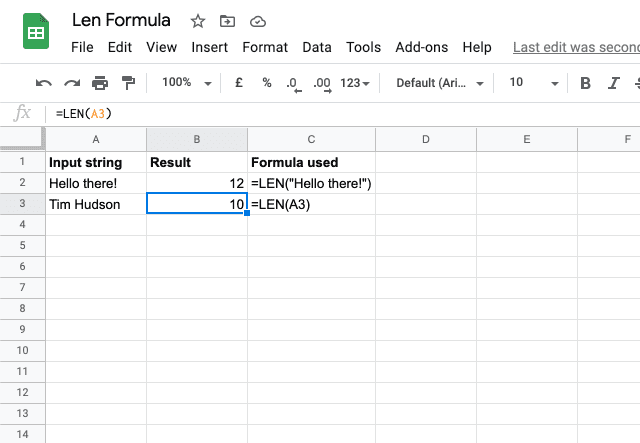This function accepts direct text values within a pair of double quotes. This is shown in the first example with the formula =LEN(“Hello there!”). It also accommodates a reference to the cell that has text value in it, as shown in the second row with the function =LEN(A3). Please note that apart from the letters, the function also counts empty spaces and special characters.

### LEN function over a range of cells

The LEN function is not limited to just one cell – it can be used to count the characters in a range of cells. To do this, however, you need to combine the LEN function with the SUMPRODUCT function.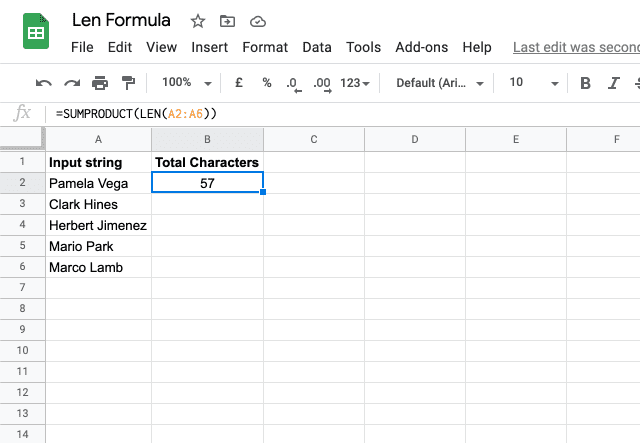I used the formula =SUMPRODUCT(LEN(A2:A6)), therefore I am able to count the total number of characters within that range.

### Separating first and last names

Simply finding the length of a text string may not be very useful depending on your situation. The real utility of the LEN function lies when it’s used in conjunction with other text functions.

As a simple example, assume that you need to extract last names from a list of names. The composite names are in this format: [first name] + [empty space] + [last name]. The names cannot be separated with the LEN function only because they are not all of the same length. The only thing you know is that there is a space between the first and last names.

We made use of the RIGHT, FIND, and LEN functions to achieve the following result. I encourage you to think through how this composite function is extracting the last names.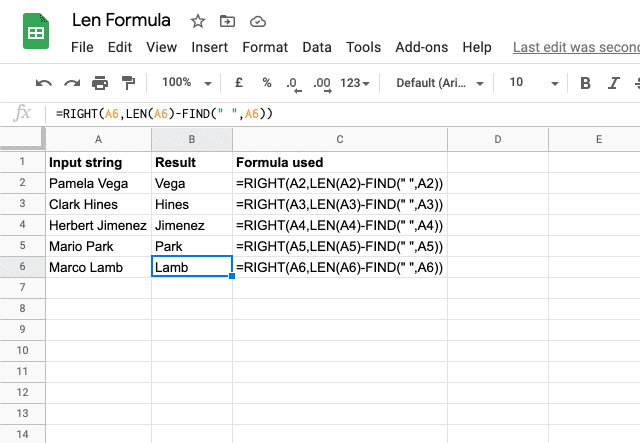Use the formula =RIGHT(A2,LEN(A2)-FIND(” “,A2)) to return the text after the space, which will always be the last name in this dataset.

Essentially, the formula uses the FIND function to identify where the space is, then subtracts that from the overall length of the string in order to use the RIGHT function to fetch the text that is on the right side of the space.

As a result, the combined function fetches the text on the right side of the space, which in this case, is always the last name!

### Excluding spaces

You’ve now seen the LEN function put to use in its basic form as well as a more complex application. But what if you want to count the number of characters without including spaces? Don’t worry, there’s a way to do that! In the example below, I show two different versions of excluding spaces.

The first (column C) excludes “additional” spaces only — extra spaces that are at the beginning or end of the string, or double spaces within the string. It still counts a single space between words. This is done using the TRIM function, which gets rid of these additional spaces. So simply type =LEN(TRIM(A2)) to count the characters in cell A2 without worrying that there are hidden additional spaces that mess up your count.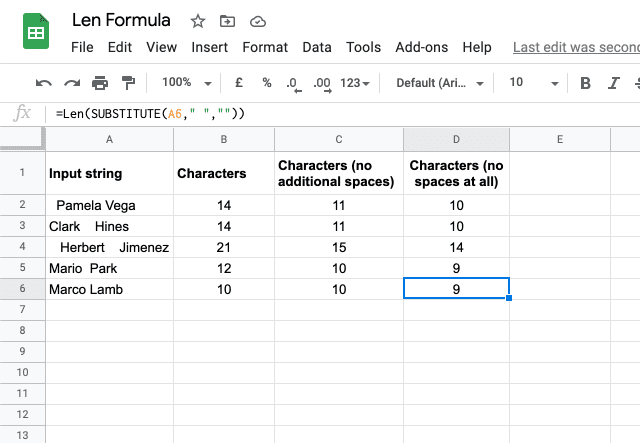When you don’t want to count any spaces at all, even between words, use the following example.

In column D, I use the SUBSTITUTE function to turn all spaces into an empty string, essentially deleting them. Then the LEN function counts up the characters of this altered string, which doesn’t have any spaces for the function to count. This is done by typing =Len(SUBSTITUTE(A2,” “,””)).

### Counting specific characters

Now that you’ve been introduced to the SUBSTITUTE function, I’ll use it again in a slightly more complicated way. This time, I created a function that will count the number of occurrences of a specific character.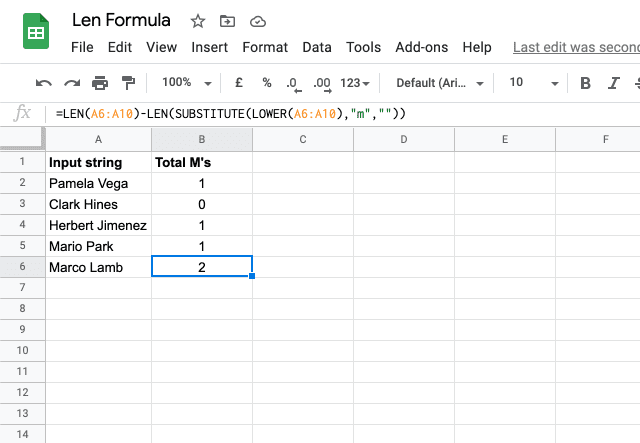In the image above, the function is counting how many m’s are in each text cell. This is done with the formula =LEN(A2:A6)-LEN(SUBSTITUTE(LOWER(A2:A6),”m”,””)).

Essentially, it is using the same logic as the last example, where we substituted the spaces with blank text strings. In this example, we substitute the letter m with a blank text string, and the difference between the length of this altered text string and the original string is the number of m’s.

We also use the LOWER function to convert all the m’s into lower case – otherwise any capital M’s wouldn’t be counted. If you only want to count upper or lower case letters, just leave out the LOWER function.

### Counting words

By now you hopefully have a good grasp on how to count characters in a variety of scenarios. Now I’ll show you how to count the number of words that are contained within a text string. Again, this method uses the SUBSTITUTE function. The full formula here is =LEN(TRIM(A2))-LEN(SUBSTITUTE((A2),” “,””))+1.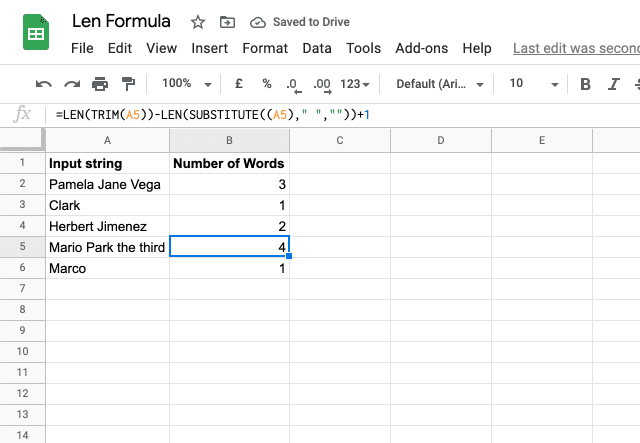The logic in this is fairly straightforward: if every word has a space in between, then count the number of spaces (and add one to represent the first word, which doesn’t have a space before it) you arrive at the number of words in a string.

This is again done by substituting the spaces for an empty string, then subtracting the length of that string from the length of the unaltered string. The TRIM function gets rid of any extra hidden spaces in the text.

## LEN function

That’s how you use the LEN function in Google Sheets! Looking for more spreadsheet tutorials and tips? Check out our other posts below!